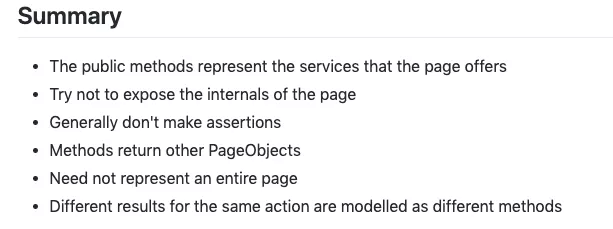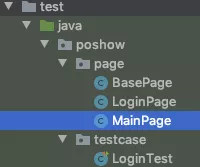# PageObject（PO）设计模式在 UI 自动化中的实践总结（以 QQ 邮箱登陆为例）

## PageObject（PO）设计模式在 UI 自动化中的实践总结（以 QQ 邮箱登陆为例）

1. 认识 PageObject1.1 PO首次提出

PO的思想最早是2013年由IT大佬Martin Flower提出的：

— 没错，就是他 —

1.2 PO原则解读对官方的原则进行解读，我们可以得到如下的信息：

##### 1.2.1 方法意义
• 用公共方法代表UI所提供的功能

• 方法应该返回其他的PageObject或者返回用于断言的数据

`要么返回一个页面` ，这个页面可以是当前页(因为可能还要在这个页面进行其他操作)，可以是其他页面(我们操作某个方法后很可能会跳转到另一个页面进行下一步操作)；

`要么返回需要断言的值` ，测试用例总归有预期结果的对吧，那么最后肯定要有方法返回一个值，用来给我们做断言，来判断用例执行是否符合预期结果。

`不要返回null或者写一个void没有返回值的方法` 这样的方法没有意义，既不能为下一步操作创造条件，也不能为用例的断言提供结果。

• 同样的行为不同的结果可以建模为不同的方法

`同样的行为` ： 无论输入的账号密码正确与否，都是按照 `输入账号密码``点击登录` 这样的行为去操作

`不同的结果` ：账号密码错误和正确得到的登录响应一定是不同的。

`建模为不同的方法` ：对于登录页来说，就可以根据登录信息正确与否建模出 `正确登录``账号错误登录``密码错误登录` 等方法了

• 不要在方法内加断言

##### 1.2.2 字段意义
• 不要暴露页面内部的元素给外部

• 不需要建模UI内的所有元素

1.3 PO的做法和优点

• 以页面为单位独立建模
• 隐藏实现细节
• 本质是面向接口编程
##### 1.3.2 基于POM的用例组织结构
• page ：完成对页面的封装
• driver ：完成对Web、Android、Ios、接口的驱动
• testcase ：调用各类page完成业务流程并进行断言
• data ：配置文件和数据驱动
• utils ：其他便捷的功能封装(可选)

1.3.3 PO的优点

• 减少例如find click这类样板代码的重复
• 测试用例的可读性提高，只关心业务流程
• 测试用例可维护性提高，UI页面频繁被修改了，我们只需要去修改对应PO即可，用例无需修改

2、PO封装演示2.1 登录场景预设

2.1 登录场景预设

2.2 代码实现

1）创建基础类 `BasePage` ，初始化 `driver` ，并封装常用的元素操作方法，如 `click``sendKeys`

``````package poshow.page;
``````
``````
``````
``````import org.openqa.selenium.By;
``````
``````import org.openqa.selenium.WebDriver;
``````
``````import org.openqa.selenium.WebElement;
``````
``````
``````
``````import java.util.List;
``````
``````
``````
``````public class BasePage {
``````
``````
``````
``````    public static WebDriver driver;
``````
``````
``````
``````
``````
``````    public WebElement findElement(By by){
``````
``````        return driver.findElement(by);
``````
``````    }
``````
``````
``````
``````    public List<WebElement> finElements(By by){
``````
``````        return driver.findElements(by);
``````
``````    }
``````
``````
``````
``````    public void click(By by){
``````
``````       findElement(by).click();
``````
``````    }
``````
``````
``````
``````    public void sendKeys(By by,String context){
``````
``````        findElement(by).sendKeys(context);
``````
``````    }
``````
``````
``````
``````    public String getText(By by){
``````
``````        return findElement(by).getText();
``````
``````    }
``````
``````}
``````

2）创建 `MainPage` 类，用于登录成功后的返回页面，由于这里并未演示登录后的操作，所以类中无具体方法实现，仅作为 `loginSuccess` 后的返回对象

``````package poshow.page;
``````
``````
``````
``````public class MainPage extends BasePage{
``````
``````}
``````

3）创建 `LoginPage` 类，继承 `BasePage` 类。定义所需元素定位方式并根据操作动作（输入账号、输入密码、点击登录）将其封装成具体的业务操作方法，例如登录成功，用户名错误登录、密码错误登录等，输入的测试数据作为方法的入参传入(username,password)

``````package poshow.page;
``````
``````
``````
``````import org.openqa.selenium.By;
``````
``````import org.openqa.selenium.chrome.ChromeDriver;
``````
``````import java.util.concurrent.TimeUnit;
``````
``````
``````
``````public class LoginPage extends BasePage{
``````
``````    //定位器
``````
``````    By usernameInput = By.name("u");  //获取用户名输入框
``````
``````    By passwordInput = By.id("p");    //获取密码输入框
``````
``````    By submitLogin = By.cssSelector("#login_button"); //获取登录按钮
``````
``````    By ErrM = By.id("err_m");  //获取错误提示信息
``````
``````
``````
``````
``````
``````    public void openUrl(){
``````
``````        String url = "https://mail.qq.com/";
``````
``````        driver = new ChromeDriver();
``````
``````        driver.manage().timeouts().implicitlyWait(5, TimeUnit.SECONDS);
``````
``````        driver.get(url);
``````
``````        driver.manage().window().maximize();
``````
``````        driver.switchTo().frame("login_frame");
``````
``````
``````
``````    }
``````
``````
``````
``````    private void sleepWait(){
``````
``````        try {
``````
``````            Thread.sleep(500);
``````
``````        } catch (InterruptedException e) {
``````
``````            e.printStackTrace();
``````
``````        }
``````
``````    }
``````
``````
``````
``````    //业务方法
``````
``````
``````
``````    /*
``````
``````    登录方法
``````
``````     */
``````
``````    private void login(String username,String password){
``````
``````        findElement(usernameInput).clear();
``````
``````        findElement(passwordInput).clear();
``````
``````        sendKeys(usernameInput,username);
``````
``````        sendKeys(passwordInput,password);
``````
``````        click(submitLogin);
``````
``````    }
``````
``````
``````
``````    /*
``````
``````      成功登录
``````
``````     */
``````
``````    public MainPage loginSuccess(String username,String password){
``````
``````        login(username,password);
``````
``````        return new MainPage();
``````
``````    }
``````
``````
``````
``````
``````
``````    /*
``````
``````     密码错误登录
``````
``````     message:你输入的帐号或密码不正确，请重新输入。
``````
``````     */
``````
``````    public String loginWithErrPassword(String username,String password ){
``````
``````        login(username,password);
``````
``````        sleepWait();
``````
``````        return getText(ErrM);
``````
``````    }
``````
``````
``````
``````    /*
``````
``````    账号为空登录
``````
``````    你还没有输入帐号！
``````
``````     */
``````
``````    public String loginWithErrUsername(String username,String password){
``````
``````        login(username,password);
``````
``````        sleepWait();
``````
``````        return getText(ErrM);
``````
``````
``````
``````    }
``````
``````
``````
``````    /*
``````
``````    密码为空登录
``````
``````     */
``````
``````    public String loginWithoutPassword(String username,String password){
``````
``````        login(username,password);
``````
``````        sleepWait();
``````
``````        return getText(ErrM);
``````
``````    }
``````
``````}
``````

4）最后创建 `LoginTest` 测试类，编写测试用例；用例的编写更接近于人的行为，人想要登录邮箱，只需要依靠用户名和密码完成登录的行为即可，无需关注具体的输入框和登录按钮是如何定位，如何进行输入点击的。并在用例中加入断言进行判断。

``````package poshow.testcase;
``````
``````
``````
``````import org.junit.jupiter.api.*;
``````
``````import poshow.page.LoginPage;
``````
``````import static org.hamcrest.CoreMatchers.equalTo;
``````
``````import static org.hamcrest.MatcherAssert.assertThat;
``````
``````
``````
``````@TestMethodOrder(MethodOrderer.OrderAnnotation.class)
``````
``````public class LoginTest {
``````
``````
``````
``````    LoginPage loginPage = new LoginPage();
``````
``````
``````
``````   @BeforeAll
``````
``````   static void openUrl(){
``````
``````        new LoginPage().openUrl();
``````
``````    }
``````
``````
``````
``````    @Test
``````
``````    @DisplayName("密码错误登录")
``````
``````    @Order(1)
``````
``````    void loginWithErrPassword(){
``````
``````        String username = "376057520";
``````
``````        String password = "123456";
``````
``````        String expectedErrM = "你输入的帐号或密码不正确，请重新输入。";
``````
``````
``````
``````        String errM = loginPage.loginWithErrPassword(username, password);
``````
``````        assertThat(errM,equalTo(expectedErrM));
``````
``````    }
``````
``````
``````
``````    @Test
``````
``````    @DisplayName("账号错误登录")
``````
``````    @Order(2)
``````
``````    void loginWithErrUsername(){
``````
``````        String username = "111";
``````
``````        String password = "123456";
``````
``````        String expectedErrM = "请输入正确的帐号！";
``````
``````
``````
``````        String errM = loginPage.loginWithErrUsername(username, password);
``````
``````        assertThat(errM,equalTo(expectedErrM));
``````
``````    }
``````
``````
``````
``````    @Test
``````
``````    @DisplayName("空密码登录")
``````
``````    @Order(3)
``````
``````    void loginWithoutPassword(){
``````
``````        String username = "376057520";
``````
``````        String password = "";
``````
``````        String expectedErrM = "你还没有输入密码！";
``````
``````
``````
``````        String errM = loginPage.loginWithoutPassword(username, password);
``````
``````        assertThat(errM,equalTo(expectedErrM));
``````
``````    }
``````
``````
``````
``````    @Test
``````
``````    @DisplayName("正确登录")
``````
``````    @Order(4)
``````
``````    void logSuccess(){
``````
``````       String username = "376057520";
``````
``````       String password = "xxx";
``````
``````       loginPage.loginSuccess(username,password);
``````
``````    }
``````
``````
``````
``````}
``````
``````
``````

5）整体结构展示：2.3 运行效果3、补充说明3.1 用例设计

• case尽量保持独立
• suite体系管理用例的顺序
• 不要把大量的业务校验逻辑放到UI自动化测试里， UI主要校验的是用户交付，操作流程，样式、数据、兼容性。
• 与接口测试合理的分工 #### 3.2 补充说明 以上仅仅是为了演示PO而举的一个简单的demo，实际上还有很大的优化空间：
• 常用元素操作方法可以进一步封装的更完善
• 可封装常用的操作util类，例如滑动
• 特定元素的等待采用显示等待
• 登录用例可以利用参数化来以数据驱动的方式完成，使用例代码更简洁易懂
• `PO` 代码和 `testcase` 代码可以分开， `test` 下只放 `case` 代码

1 Like